Set (=)  versus  SetDelayed (:=)

Alan Hayes provided an example where (  func[x_]=  ) instead of

( func[x]:=  ) should be used.  If ( func[x_]:=  ) was used in this example

the least squares fit would be computed  again for every value of (x).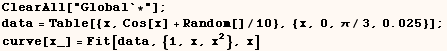In the following line Alan uses "Epilog->Point/@data"  to ensure the data
points

are drawn along with a Plot of the curve.  For this application it makes

little difference whether Epilog or Prolog is used.In the following example (expr) is not a polynomial in (x) until (expr) is
evaluated.  So we have to use Set (=) to have the function perform as
intended.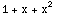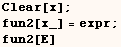In the next example the right hand side can't evaluate until x is given a
numeric value, so we have to use Set (=).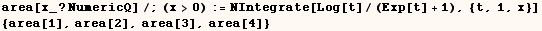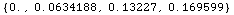In the next example evaluation of (deriv1[expr]) starts with substituting the
value of (expr) for (x) in the definition of deriv1[x_].  Next the Derivative
is evaluated, and all is well as (expr) does not have a numeric value.  When
the argument of ( deriv1 )  is a numeric value the value is substituted for
(x) in the definition of (deriv1), and a Derivative with respect to a
constant is not defined.  As a result (deriv1) doesn't work for numeric
arguments.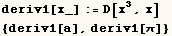In the next cell the definition of (deriv2) differs from the definition of
(deriv1) in that Set (=) is used rather than SetDelayed (:=).  Here the
Derivative is only evaluated  when (deriv2) is defined.  The new function
(deriv2) gives the expected result for symbolic or numeric arguments.Notice the definition of (deriv1) involves the Derivative operation, but the
definition of (deriv2) does not.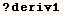Global`deriv1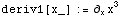Global`deriv2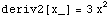In the next two lines a Cumulative Distribution Function is defined.  For
CDF1 SetDelayed (:=) must be used because NIntegrate can not evaluate until
we have a numerical value for both limits (upper and lower).  On the other
hand CDF2 is defined using Set (=).  In this case SetDelayed (:=) could have
been used but this would be far less efficient.  When CDF2 is defined using
Set (=) the symbolic integration is only performed when CDF2 is defined.  If
CDF2 was defined using SetDelayed (:=) the symbolic integration would be
performed every time CDF2[_] is evaluated.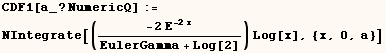The next example is from the FAQ section of the Wolfram Research web page.
When ( f1[n_] ) is defined using Set (=)  Expand has no effect because Expand
makes no change if the exponent is not a positive integer.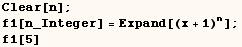On the other hand ( f2[n_] ) is defined using SetDelayed (:=), and

Expand does not evaluate until the exponent has an integer value.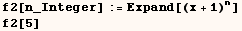A different perspective on explaining Set versus SetDelayed is give in the  notebook posted at  http://library.wolfram.com/infocenter/MathSource/425/

A warning about named patterns in lhs when using (lhs=rhs)

One must be careful about using (lhs=rhs) when (lhs) includes named patterns
and the pattern name is needed in (rhs).  Named patterns can include (x_),
(x__), (opts___), (m_:4) (j_Integer), (t_?Positive), (n_.) and many other
forms. When the variable used to name the pattern is used in (rhs) the global
value of the variable is used in (rhs).  The next two cells demonstrate the
problem.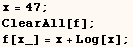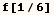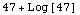Above we see the global value of (x) is used instead of 0.125 to compute  f[x].  An easy way to avoid the problem is to clear any symbols used to name  patterns when the patterns are needed on the left side.  Below we can be sure  that the definition will work as intended because any Global values were  cleared from x.  This obviously requires that the variables used to name  patterns have no variables that you care about.  Another solution that  doesn't have this limitation is available from my MathSource item at  http://library.wolfram.com/database/MathSource/425.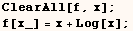However, you can use the symbol used to name a pattern variable on the left
side of (=) with out problems.  Below the global value of (x) doesn't prevent
us from getting the intended result.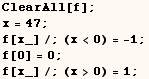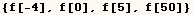Evaluation of (lhs=rhs)

The next cell causes (g) to evaluate to (h) and causes (h) to evaluate to 9.
As a result both (g) and (h) evaluate to 9.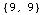In the next cell (g=0) is evaluated and the value of zero is assigned to (g)
without letting (g) evaluate.  As a result (g) evaluates 0 but (h) still
evaluates to 9.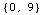From the examples above it appears (lhs) in (lhs=rhs) doesn't evaluate and
that is the case if MatchQ[Unevaluated[lhs],_Symbol] would return True.
However, it isn't that simple if Unevaluated[lhs] isn't a Symbol. To
demonstrate this consider the next cell where both (h) and (g) return
9.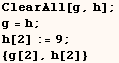Now when the next cell is evaluated (g[1+Tan[π/2]]) partially evaluates
prior to assigning the value 0 to something. First (g) evaluates to (h). Then
(1+Tan[π/4]) evaluates to (2). The last thing to happen is that 0 is
assigned to h.  The first argument is held in the sense that h doesn't
evaluate to 9. If that happened this example would end up with 9=0 which is
nonsense. A user defined function with the (HoldFirst) attribute will not
work this way unless complicated definitions are used to ensure that it does.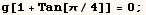Now when g is evaluated (g) evaluates to (h) and h evaluates to 0.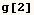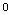Below we see that the new assignment is associated with (h) not with (g).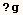Global`g

 g = h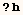Global`h

 h = 0

Created by Mathematica  (May 16, 2004)

Back to Ted’s Tricks index page